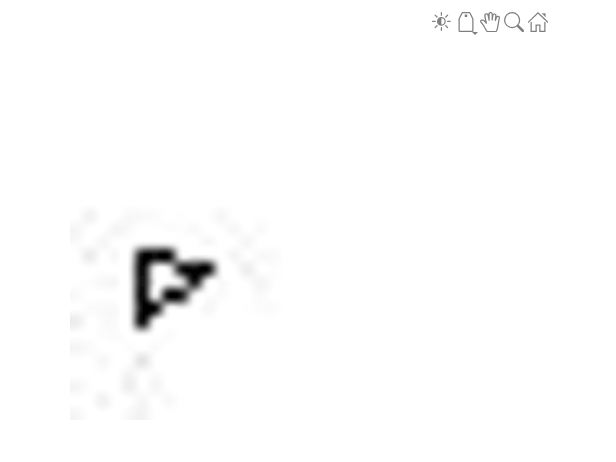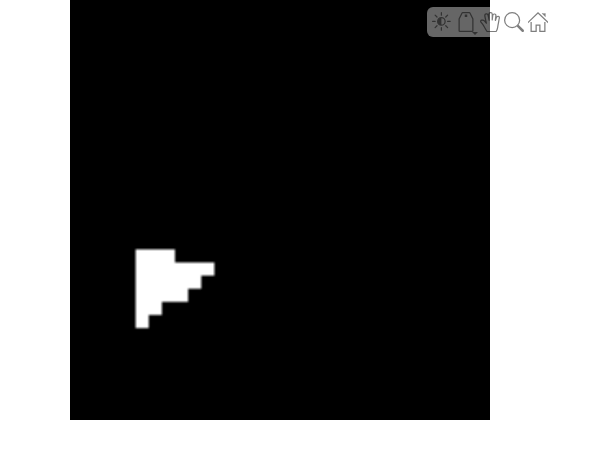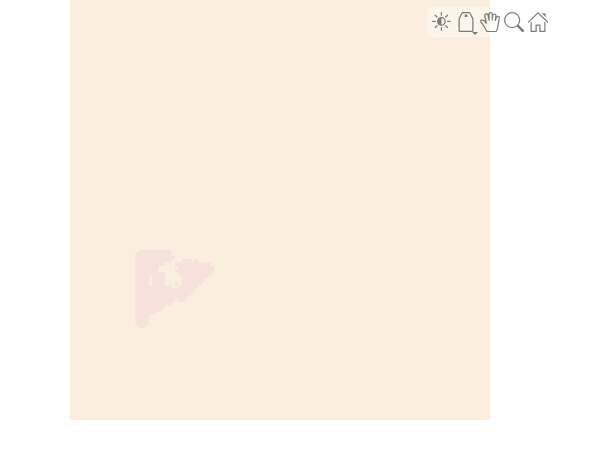Calculate Segmentation Metrics in Block-Based Workflow

This example shows how to calculate the semantic segmentation confusion matrix for individual blocks in a blockedImage object, then calculate global and block segmentation metrics.

Load a pretrained network that performs binary segmentation of triangles against a background.

The triangleImages data set has 100 test images with ground truth labels. Define the location of the data set.

dataSetDir = fullfile(toolboxdir('vision'),'visiondata','triangleImages');

Define the location of the test images.

testImagesDir = fullfile(dataSetDir,'testImages');

Read three test images. Resize each image by a factor of four, convert it to data type double, then create a blockedImage object. A blockedImage supports block-based image processing workflows.

numImages = 3;
for idx = 1:numImages
im = imread(fullfile(testImagesDir,['image_' '00' num2str(idx) '.jpg']));
im = imresize(im,4);
testImages(idx) = blockedImage(im);
end

Display the first test image.

bigimageshow(testImages(1))Define the location of the ground truth labels.

testLabelsDir = fullfile(dataSetDir,'testLabels');

Define the class names and their associated label IDs.

classNames = ["triangle","background"];
labelIDs   = [255 0];

Read in the ground truth labels for each test image. Create a blockedImage object from each ground truth label.

for idx = 1:numImages
gtLabel = imread(fullfile(testLabelsDir,['labeled_image_' '00' num2str(idx) '.png']));
gtLabel = imresize(gtLabel,4,'nearest');
groundTruthImages(idx) = blockedImage(gtLabel);
end

Display the first ground truth image.

bigimageshow(groundTruthImages(1))For each test image, use the apply function to process each block. The apply function performs the operations specified by the helper function segmentAndCalculateBlockMetrics, which is defined at the end of this example. The function performs semantic segmentation of each block and calculates the confusion matrix between the predicted and ground truth labels.

blockSize = [32 32];
datasetConfMat = table;
for idx = 1:numImages
[segmentedImages(idx),blockConfMatOneImage] = apply(testImages(idx), ...
@(block,labeledImageBlock) segmentAndCalculateBlockMetrics(block,labeledImageBlock,net,classNames,labelIDs), ...

% Read all the block results of an image and update the image number
blockConfMatOneImageDS = blockedImageDatastore(blockConfMatOneImage);
blockConfMat = struct2table([blockConfMat{:}]);
blockConfMat.ImageNumber = idx.*ones(height(blockConfMat),1);
datasetConfMat = [datasetConfMat;blockConfMat];
end

Display the first segmented image.

bigimageshow(segmentedImages(1))Evaluate the data set metrics and block metrics for the segmentation.

[metrics,blockMetrics] = evaluateSemanticSegmentation(datasetConfMat,classNames,'Metrics','all');
Evaluating semantic segmentation results
----------------------------------------
* Selected metrics: global accuracy, class accuracy, IoU, weighted IoU.
* Processed 3 images.
* Finalizing... Done.
* Data set metrics:

GlobalAccuracy    MeanAccuracy    MeanIoU    WeightedIoU
______________    ____________    _______    ___________

0.95428          0.82739       0.69927      0.92533

Calculate the Jaccard score for all images.

jaccardSimilarity = metrics.ImageMetrics.MeanIoU
jaccardSimilarity = 3×1

0.7664
0.7277
0.6538

Supporting Function

The segmentAndCalculateBlockMetrics function performs semantic segmentation of a single block then calculates the confusion matrix of the predicted and ground truth labels.

function [outputLabeledImageBlock,blockConfMatPerBlock] = segmentAndCalculateBlockMetrics(block,labeledImageBlock,net,classNames,labelIDs)

outputLabeledImageBlock = semanticseg(block.Data,net);

% Convert the ground truth labels to categorical
labeledImageBlock = categorical(labeledImageBlock,labelIDs,classNames);
confusionMatrix = segmentationConfusionMatrix(outputLabeledImageBlock,labeledImageBlock);

% blockConfMatPerBlock is a struct with confusion matrices, image number
% and blockInfo. Use the struct with evaluateSemanticSegmentation to
% calculate metrics and aggregate block-based results.
blockConfMatPerBlock.ConfusionMatrix = confusionMatrix;
blockConfMatPerBlock.ImageNumber = block.ImageNumber;

blockInfo.Start = block.Start;
blockInfo.End = block.End;
blockConfMatPerBlock.BlockInfo = blockInfo;
end